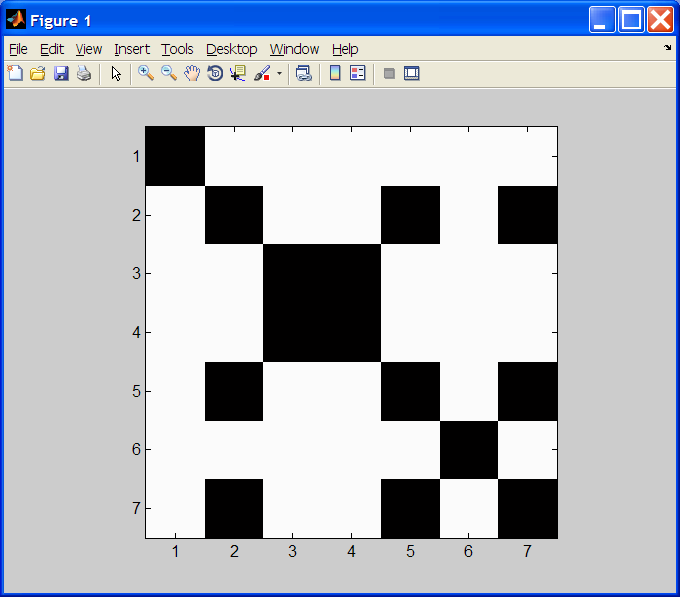Homework #7CS 107 - Introduction to Scientific ComputationHomework #7

Due: Wednesday 10/16 at the beginning of class

Submit from your .m file directory using the command:
submit107 hw7

## Bioinformatics Exercises

1. RNA Transcription: DNA and RNA consist of sequences of nucleotides.  DNA nucleotides each contains one of the following bases: adenine, cytosine, guanine, and thymine, abbreviated A, C, G, and T, respectively.   In bioinformatics, a DNA sequence is often represented as a string made up of these abbreviated base names (e.g. "GATTACA").  RNA uses uracil (U) in place of thymine (T).  When RNA is transcribed from DNA, the result is a "copy" of the DNA sequence with uracil (U) substituted in the RNA for each occurrence of thymine (T) in the DNA.  Create a function `transcribe.m` that takes a string of DNA bases, and returns the corresponding string of transcribed RNA bases.  For example:

```>> transcribe('GATTACA')

ans =

GAUUACA```

2. RNA Codon Translation: Exercise 8.7.  Create a  function `rna2amino` that takes two arguments, an RNA nucleotide sequence string and a starting index, and returns the amino acid sequence string that would be translated.

Hint:  One approach makes use of a switch/case statement to map sets of codons to the smaller number of acids/'Stop'.  Another approach would be to start your definition as follows:

```function res = rna2amino(bases, startIndex)
acids = ['Phe'; 'Ser'; 'Tyr'; 'Cys'; ...
'Phe'; 'Ser'; 'Tyr'; 'Cys'; ...
'Leu'; 'Ser'; 'Stp'; 'Stp'; ...
'Leu'; 'Ser'; 'Stp'; 'Trp'; ...
'Leu'; 'Pro'; 'His'; 'Arg'; ...
'Leu'; 'Pro'; 'His'; 'Arg'; ...
'Leu'; 'Pro'; 'Gln'; 'Arg'; ...
'Leu'; 'Pro'; 'Gln'; 'Arg'; ...
'Ile'; 'Thr'; 'Asn'; 'Ser'; ...
'Ile'; 'Thr'; 'Asn'; 'Ser'; ...
'Ile'; 'Thr'; 'Lys'; 'Arg'; ...
'Met'; 'Thr'; 'Lys'; 'Arg'; ...
'Val'; 'Ala'; 'Asp'; 'Gly'; ...
'Val'; 'Ala'; 'Asp'; 'Gly'; ...
'Val'; 'Ala'; 'Glu'; 'Gly'; ...
'Val'; 'Ala'; 'Glu'; 'Gly'];```
This is a 64x3 character array that has the take amino acid entries left-to-right, top-to-bottom.  Suppose you used strfind('UCAG', base) - 1, to assign an individual base a number (e.g. strfind('UCAG', 'A') - 1 2).  Consider, for a given codon, how the change of a single base's number in a single position (holding all others fixed) affects the index of the desired acid.  Form a mathematical expression from the codon's three base numbers to the acid (converting a 'Stp' result to 'Stop').

3. Dot Plot:  A dot plot is a means of visually comparing similarity of two base sequences.  Given two strings s1 and s2 of bases, coordinate (i, j) of the plot is black if s1(i) == s2(j), and is white otherwise.  NOTE: Strings s1 and s2 may have different lengths.  We'll accomplish this by building a 2D array (i.e. a matrix) x of 0's and 1's, corresponding to mismatches and matches, respectively.  We plot them as follows:

```colormap(gray(2));
image(2 - x);
axis ij;
axis square;```

For example,

`dotPlot('gattaca','gattaca')`

yieldsCreate function `dotPlot` that takes two base sequence strings, computes the dotplot data matrix, plots it as above, and returns the dotplot matrix.